# Filling a rigid tire from a pressure regulated compressed air line

• I
• erobz
I do not want to get into an argument about this.In summary, it is possible for air mass momentum to overshoot equality. If you connect two tanks with a pipe, equilibrium will never be reached in finite time.
Ok, I think I found another route. It's the same type of derivation as (10) ( from Reynolds Transport Theorem ) but its known as the "Momentum Equation" in fluid mechanics ( It is Newtons Second Law for fluid systems ). Which is basically how I had started this whole thing before I led everyone on the wild goose chase!

$$\sum F = \frac{d}{dt} \int_{cv} \boldsymbol v \rho d V\llap{-} +\int_{cs} \boldsymbol v \rho \boldsymbol V \cdot d \boldsymbol A$$

I'm not sure why there are different symbols for the velocity ## \boldsymbol v, \boldsymbol V ## , but that is how it is in the textbook.

Anyhow the control volume is the pipe between the tanks

$$\sum F = P_1 A - P_2 A -\tau \pi D l$$

Momentum Accumulation in the Control Volume:

Incompressible flow with constant cross sectional area ##A ## implies that the velocity is not varying along the length of the pipe and can come outside of the integral along with the density:

$$\frac{d}{dt} \int_{cv} \boldsymbol v \rho d V\llap{-} = \frac{d}{dt} \rho v A \int_{cv} dl = \rho A l \frac{dv}{dt}$$

The second integral is the Net Efflux of Momentum across the control surface, and because of my assumptions of uniform velocity distribution, incompressibility, and constant cross sectional area it is identically 0:

$$\int_{cs} \boldsymbol v \rho \boldsymbol V \cdot d \boldsymbol A = 0$$

That implies we have:

$$\sum F = P_1 A - P_2 A -\tau \pi D l = \rho A l \frac{dv}{dt}$$

We've moved ## P_1, P_2 ## from the tank surface to the inlet and outlet of the pipe it follows that:

$$P_1 = \rho g z = \rho g \frac{1}{A_1} \left( V\llap{-} - {V\llap{-}}_p - A_2 h \right)$$

$$P_2 = \rho g h$$

$$v_p = \frac{A_2}{A_p} \dot h$$

That means that finally, and I hope to the satisfaction of all involved in the hunt for oscillation, exponential decay we arrive at:

$$\rho g \left(\frac{1}{A_1} \left( V\llap{-} - {V\llap{-}}_p - A_2 h \right) \right) - \rho g h - \tau \pi D l = \rho A l \ddot h$$

I'm not going to bother to simplify it more at this time.

It's a Second Order,Linear ODE of the Non-Homogeneous variety.

erobz said:
$$\rho g \left(\frac{1}{A_1} \left( V\llap{-} - {V\llap{-}}_p - A_2 h \right) \right) - \rho g h - \tau \pi D l = \rho A l \ddot h$$

I'm not going to bother to simplify it more at this time.

It's a Second Order,Linear ODE of the Non-Homogeneous variety.
But if I understand it correctly, the solution is just sinusoidal!

•erobz
erobz said:
I think I've found what you are looking for in the latest approach.

$$\rho g \left(\frac{1}{A_1} \left( V\llap{-} - {V\llap{-}}_p - A_2 h \right) \right) - \rho g h - \tau \pi D l = \rho A l \ddot h$$

Unless you explain what the variables all are and how they depend on each other I can't make anything out of this. My comment assumes this was a differential equation in the variable ##h## and the other stuff was constants. If that is not the case then show the dependence on ##h## please.

bob012345 said:
But if I understand it correctly, the solution is just sinusoidal!
actually, I don't think so.

$$\rho g \left(\frac{1}{A_1} \left( V\llap{-} - {V\llap{-}}_p - A_2 h \right) \right) - \rho g h > 0$$

Then we bring it over to the RHS:

$$\ddot h - k h = -b$$

The solution to that ( I believe ) is:

$$h = c_1 e^{kt} + c_2 e^{-kt} + C$$

You're going to try and make me solve it!Last edited:
bob012345 said:
$$\rho g \left(\frac{1}{A_1} \left( V\llap{-} - {V\llap{-}}_p - A_2 h \right) \right) - \rho g h - \tau \pi D l = \rho A l \ddot h$$

Unless you explain what the variables all are and how they depend on each other I can't make anything out of this. My comment assumes this was a differential equation in the variable ##h## and the other stuff was constants. If that is not the case then show the dependence on ##h## please.

Thats is all explained in post #15

https://www.physicsforums.com/threa...ated-compressed-air-line.1015542/post-6636284

bob012345 said:
$$\rho g \left(\frac{1}{A_1} \left( V\llap{-} - {V\llap{-}}_p - A_2 h \right) \right) - \rho g h - \tau \pi D l = \rho A l \ddot h$$

Unless you explain what the variables all are and how they depend on each other I can't make anything out of this. My comment assumes this was a differential equation in the variable ##h## and the other stuff was constants. If that is not the case then show the dependence on ##h## please.

Yeah, its a Second order ODE with constant coefficients. It looks like it would be of the form from how I have it written:

$$\ddot h + k h = -b$$

giving you the oscillating solution, but its actually ## z > h ##

So its actually of the form:

$$\ddot h - k h = -b$$

Giving the exponential solution.

erobz said:
Yeah, its a Second order ODE with constant coefficients. It looks like it would be of the form from how I have it written:

$$\ddot h + k h = -b$$

giving you the oscillating solution, but its actually ## z > h ##

So its actually of the form:

$$\ddot h - k h = -b$$

Giving the exponential solution.
Then the equation needs correcting to account for that because just solving what is written the solution oscillates. But the important point is you now agree it is an exponential solution as @Baluncore pointed out.

bob012345 said:
Then the equation needs correcting to account for that because just solving what is written the solution oscillates. But the important point is you now agree it is an exponential solution as @Baluncore pointed out.
maybe I did something wrong, I'll clean it up.

erobz said:
maybe I did something wrong, I'll clean it up.
I still recommend for the water tank case to take a step back and simplify. Assume everything happens quasi-statically. Maybe do a simple case of water leaking from one tank first. Then add the pressure of the second tank opposing that flow.

bob012345 said:
Then the equation needs correcting to account for that because just solving what is written the solution oscillates. But the important point is you now agree it is an exponential solution as @Baluncore pointed out.
lol, but now that I've done something wrong I'm not sure! Don't hate me...

•bob012345
erobz said:
lol, but now that I've done something wrong I'm not sure! Don't hate me...
Don't despair! If you have no viscous effects I think you should get an oscillation. Now if you add viscosity you should get a damped oscillation as @Baluncore discussed above. If it is critically damped you get the exponential decay.

bob012345 said:
Don't despair! If you have no viscous effects I think you should get an oscillation. Now if you add viscosity you should get a damped oscillation as @Baluncore discussed above. If it is critically damped you get the exponential decay.

Ohhh. I'm dispairing! Viscous effects are in the model!

These are the tanks and relevant parameters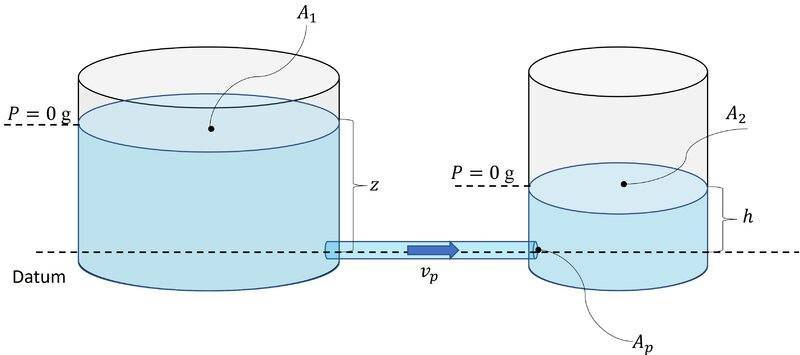This is the control volume inside the pipe joining the tanks: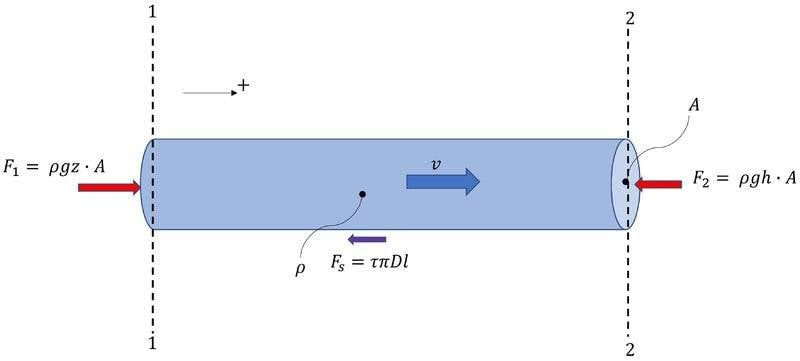The tanks are removed and replaced with their effective external forces on the control volume

## F_{shear} = \tau \pi D l## this is the viscous force that resists flow

## F_1 = \rho g z A ## hydrostatic pressure from tank 1 acting on ##A##

## F_2 = \rho g h A ## hydrostatic pressure from tank 2 acting on ##A##

## \rho A l ## is the mass of the control volume

I have that:

$$\rho A l \frac{dv}{dt} = \rho g z A - \rho g h A - \tau \pi D l \tag{1}$$

The total Volume of the system ## V\llap{-} ## is given by:

$$A_1 z + A_2 h + {V\llap{-}}_{pipe} = V\llap{-}$$

$$\Downarrow$$

$$z = \frac{ V\llap{-} - {V\llap{-}}_{pipe} }{ A_1} - \frac{A_2}{A_1} h \tag{2}$$

$$\Downarrow$$

$$\dot z = -\frac{A_2}{A_1} \dot h \tag{3}$$

and applying continuity between the pipe and tank 2:

$$v A = A_2 \dot h \tag{4}$$

So all together we have that:

$$\rho l A \frac{ A_2}{A} \ddot h = \rho g A \left( z - h \right) - \tau \pi D l \tag{5}$$

The criterion here is that

$$z \geq h$$

hence ## z - h \geq 0 ## must be satisfied for the solution to hold So whatever this term is, it is positive on the RHS and must be negative on the LHS of (5).

This implies that there are bounds on ## h ## determined by (2)?

Other than that, I have no idea how to resolve this if its incorrect? Is there something that happens when the particular solution is added to the homogenous solution? I'm not seeing it if that is the case. Certainly stepping into the Twilight Zone a little bit here...

Last edited:
It looks to me that the viscous effect you included is just a constant. In a LRC damped oscillator the resistance R is multiplied by ##\dot{I}##. Maybe the shear force needs an ##\dot{h}##? Did you include Poiseuille's Law for fluids? Also, it might be easier to make the variable ##z-h##.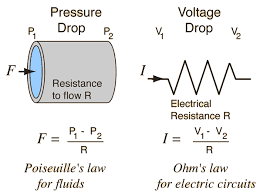https://courses.physics.ucsd.edu/2015/Summer/physics2cl/documents/Phys2CL_Exp2_2010.pdf

Last edited:
•erobz
bob012345 said:
It looks to me that the viscous effect you included is just a constant. In a LRC damped oscillator the resistance R is multiplied by ##\dot{I}##. Maybe the shear force needs an ##\dot{h}##?
I think you must be correct.

$$\tau_o = -\mu \left. \frac{dV}{dr} \right|_{r_o}$$

Where

## \mu ## is the kinematic viscosity of the flow

## r ## is the distance from the pipe centerline normal to the flow velocity distribution

## \left. \frac{dV}{dr} \right|_{r_o} ## this bit is the evaluation of that flow distribution at the pipe walls.

for laminar flow this is going to have a linear proportionality "##\varphi V##". For other flow distributions it varies.

I was allowing myself to be fooled once again by a derivation assuming steady flow ... where the authors weren't integrating anything, and they casully dropped ## \tau_o ## in there as if it were a constant... my bad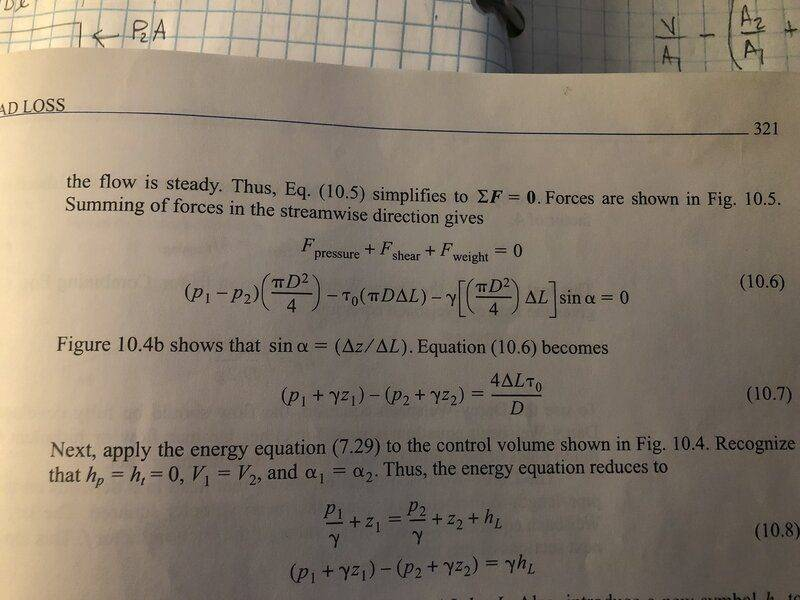Last edited:
•bob012345
What book is that? It's most likely correct for the problem it is doing which of course may be a bit different than yours.

Engineering Fluid Mechanics - Ninth Edition. Yeah, it's fine for what it is about to do in the rest of the derivation. I just didn't catch that steady flow assumption..., so for what they are doing (Deriving the Darcy Weisbach Equation for head loss in a pipe) the fact that ## \tau ## is actually a function of ##V## is not relevant at that point. They explain ## \tau ## a few pages later

Derivation grabbing...guilty as charged!

•bob012345
$$\rho l A \frac{ A_2}{A} \ddot h = \rho g A \left( \frac{ V\llap{-} - {V\llap{-}}_{pipe} }{ A_1} - \left( \frac{A_2}{A_1} + 1 \right) h \right) - \mu \pi D l \frac{A_2}{A} \dot h \tag{6}$$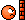Thank you ( All ) for your continued skepticism!

•bob012345
erobz said:
$$\rho l A \frac{ A_2}{A} \ddot h = \rho g A \left( z - h \right) - \mu \pi D l \frac{A_2}{A} \dot h \tag{6}$$Thank you ( All ) for your continued skepticism!
Great but one thing is what about ##z##? I suspect you can frame this in terms of ##z-h## as the variable in the equation. Also, is it ##\frac{ A_2}{A}## or ##\frac{ A_2}{A_1}##?

bob012345 said:
Great but one thing is what about ##z##? I suspect you can frame this in terms of ##z-h## as the variable in the equation. Also, is it ##\frac{ A_2}{A}## or ##\frac{ A_2}{A_1}##?
I edited it and put it all in terms of ##h##

Its ## A ## ( from the Area of the pipe) and there is some cancelation I can do...

•bob012345
bob012345 said:
Great but one thing is what about ##z##? I suspect you can frame this in terms of ##z-h## as the variable in the equation.
$$\rho l A_2 \ddot h = \rho g A \left( z - h \right) - \mu \pi D l \varphi \dot h \tag{5}$$

If we do the following substitution as you suggest from (5):

$$\lambda = z-h \implies \dot \lambda = \dot z - \dot h$$

Then substituting

$$\dot z = -\frac{A_2}{A_1} \dot h \implies \dot h = - \frac{A_1}{A_2 + A_1} \dot \lambda \implies \ddot h = - \frac{A_1}{A_2 + A_1} \ddot \lambda$$

Putting that all back into (5):

$$-\rho l \frac{A_1 A_2}{A_2 + A_1} \ddot \lambda = \rho g A \lambda + \mu \pi D l \frac{A_1}{A_2 + A_1} \varphi \dot \lambda$$

Or:

$$\rho l \frac{A_1 A_2}{A_2 + A_1} \ddot \lambda + \mu \pi D l \frac{A_1}{A_2 + A_1} \varphi \dot \lambda + \rho g A \lambda = 0$$

I'm pretty sure this now has the solution modes @Baluncore identified quite some time ago. Thanks for going the distance on this! We'll... from the original question I'm now suspecting it's more like 1/8 the distance! However, I think I can live with that...

Last edited:
•bob012345
Going back to the original problem, if the volume of the tube is much less than the volume of the tire, the tube can be assumed to be flowing at quasi-steady state. At very long times, the tire pressure will be very close to the regulator pressure, and the flow through the tube will be laminar. From the Hagen-Poiseuille equation for laminar flow, it follows that $$\dot{m}=\frac{\rho_R\pi^2D^6}{512\mu L}(p_R-p_T)$$where ##\dot{m}## is the mass flow rate through the tube, ##\rho_R## is the gas density at the regulator, ##p_R## is the regulator pressure, and ##p_T## is the tire pressure. The rate of change of pressure in the tire is given by the ideal gas law: $$\frac{\dot{m}}{M}=\frac{V}{RT}\frac{dp_T}{dt}$$If we combine these two equations, we obtain an equation of the form: $$\frac{dp_T}{dt}=k(p_R-p_T)$$The solution to this equation is such that the difference between the regulator pressure and the tire pressure decreases as $$e^{-kt}$$So the tire pressure never quite reaches the regulator pressure, although, after a time on the order of say 4/k, it closely approaches the regulator pressure.

•bob012345 and erobz
Chestermiller said:
Going back to the original problem, if the volume of the tube is much less than the volume of the tire, the tube can be assumed to be flowing at quasi-steady state. At very long times, the tire pressure will be very close to the regulator pressure, and the flow through the tube will be laminar. From the Hagen-Poiseuille equation for laminar flow, it follows that $$\dot{m}=\frac{\rho_R\pi^2D^6}{512\mu L}(p_R-p_T)$$where ##\dot{m}## is the mass flow rate through the tube, ##\rho_R## is the gas density at the regulator, ##p_R## is the regulator pressure, and ##p_T## is the tire pressure. The rate of change of pressure in the tire is given by the ideal gas law: $$\frac{\dot{m}}{M}=\frac{V}{RT}\frac{dp_T}{dt}$$If we combine these two equations, we obtain an equation of the form: $$\frac{dp_T}{dt}=k(p_R-p_T)$$The solution to this equation is such that the difference between the regulator pressure and the tire pressure decreases as $$e^{-kt}$$So the tire pressure never quite reaches the regulator pressure, although, after a time on the order of say 4/k, it closely approaches the regulator pressure.
Well, now that we have an answer, I'm only half tempted to try and work up to it (or something like it) from first principles! Thanks Chester!

erobz said:
Well, now that we have an answer, I'm only half tempted to try and work up to it (or something like it) from first principles! Thanks Chester!
It would make a nice completion to this thread! :)

•erobz
bob012345 said:
It would make a nice completion to this thread! :)
I got to be honest bob, as much as I would like to be able to do it... upon first inspection it seems to be an entirely different beast. I can spitball some ideas on how I think the "Momentum Equation" plays out, and maybe I can be work through it with much help...I'll give it a try. Put on your skeptical hat!

•bob012345
The figure below shows the new control volume that is between the regulated pressure from the tank supply ## P_r ## and the tire at pressure ##P##. I'm applying (1) the control volume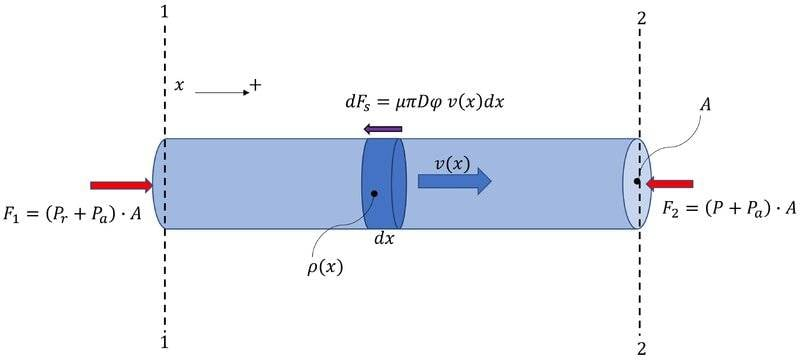$$\sum F = \frac{d}{dt} \int_{cv} \boldsymbol v \rho d V\llap{-} +\int_{cs} \boldsymbol v \rho \boldsymbol V \cdot d \boldsymbol A \tag{1}$$

##\underline{\sum F}##

Since we have from the earlier result that the Shear Force resisting the flow was porportional to the flow velocity within the variable ## \tau = \varphi v##, we now have to consider the total shear force acting the control volume as integral because the velocity is varying over the length of the tube.

$$\sum F = \left( P_r + P_a \right) A - \left( P(t) + P_a \right) A - \mu \pi D \varphi \int v(x,t) \, dx$$

Momentum Accumulation Term:

This time the only quantity that can be taken outside the integral is the cross sectional area and we can drop the vector notation.

$$\frac{d}{dt} \int_{cv} \boldsymbol v \rho d V\llap{-} = A \frac{d}{dt} \int v(x,t) \rho (x,t) dx$$

Net Efflux of Momentum Term:

I'm considering the properties ## \rho ## and ##v ## as uniformily distributed across the area of the pipe at section 1 and 2.

$$\int_{cs} \boldsymbol v \rho \boldsymbol V \cdot d \boldsymbol A = A \left( \rho_2(t) {v_2(t)}^2 - \rho_r {v_1(t)}^2 \right)$$

$$P_r A - P(t)A - \mu \pi D \varphi \int v(x,t) \, dx = A \frac{d}{dt} \int v(x,t) \rho (x,t) dx + A \left( \rho_2(t) {v_2(t)}^2 - \rho_r {v_1(t)}^2 \right)$$

As far as I can see this is step 1.

Last edited:
Instead of starting from scratch I would suggest you look at the assumptions @Chestermiller made above and start with that. Dig into what assumptions were made and why they were made. Then you can fill in the derivational details of his logical flow which was nicely laid out for you.

bob012345 said:
Instead of starting from scratch I would suggest you look at the assumptions @Chestermiller made above and start with that. Dig into what assumptions were made and why they were made. Then you can fill in the derivational details of his logical flow which was nicely laid out for you.
I haven't been able to track down the original derivation that leads to this, which is where the meat of the analysis will be.
$$\dot{m}=\frac{\rho_R\pi^2D^6}{512\mu L}(p_R-p_T)$$
I'm not sure I'm getting something here:
$$\frac{\dot{m}}{M}=\frac{V}{RT}\frac{dp_T}{dt}$$
With properties of the mass of the air inside the tire ( Temperature, Volume, Ideal Gas Constant ) fixed:

$$P = \rho R T \implies \frac{dP}{dt} = \frac{dm}{dt} \frac{RT}{V\llap{-} } \implies \dot m = \frac{V\llap{-} }{RT} \frac{dp}{dt}$$

But that's not quite what has been written ( I suspect a typo).

$$\frac{dp_T}{dt}=k(p_R-p_T)$$
Solving the resulting differential equation is straight forward with a ##u = p_R - p_T## substitution

Last edited:
erobz said:
I haven't been able to track down the original derivation that leads to this, which is where the meat of the analysis will be.

I'm not sure I'm getting something here:

With properties of the mass of the air inside the tire ( Temperature, Volume, Ideal Gas Constant ) fixed:

$$P = \rho R T \implies \frac{dP}{dt} = \frac{dm}{dt} \frac{RT}{V\llap{-} } \implies \dot m = \frac{V\llap{-} }{RT} \frac{dp}{dt}$$

But that's not quite what has been written ( I suspect a typo).
##M## is Molar mass I think and ##m## is actual mass so ##\dot{m}## is the mass flow rate. I usually write the Ideal Gas Law as ##PV= nRT## where ##n## is the number of moles. Here ##n=\large \frac{m}{M}##.

•erobz
bob012345 said:
##M## is Molar mass I think and ##m## is actual mass so ##\dot{m}## is the mass flow rate. I usually write the Ideal Gas Law as ##PV= nRT## where ##n## is the number of moles. Here ##n=\large \frac{m}{M}##.
Ahhh, yes. We typically used the Specific Gas Constant in engineering which that is "baked" into. I wasn't expecting the Chemist/Physicist standard.

Last edited:
A reality check based on the capacitor analogy.
Based on charging a capacitor from a 100 Volt source.
In one time constant, the voltage will rise to approximately 63 Volts or 63%.
At the end of one time constant, there will be 37% or 37 Volts driving the charging current.
We will start expressing the percentage charge voltage as (100% minus .63%)^N N being the number of time constants.
At the end of the Second time constant: 100V - (.37^2 x 100)V = 87V
At the end of the Fifth time constant: 100V - (.37^5 x 100)V = 99.3V
For real world applications in the field, a condition is assumed to have stabilized after five time constants.
but to continue:
At the end of 10 time constants: 100V - (.37^10 x 100)V = 99.5V
At the end of 20 time constants: 100V - (.37^20 x 100)V = 9999998V

For an exact value in place of .37, use 0.36787944117144844353582058542057 the reciprocal of E the base of natural logarithms.

Yes, I have done a lot of rounding and I have ignored a lot of factors; compressibility, temperature changes, friction, changes in atmospheric pressure, tidal effect oscillation and probably other factors.
Does it matter? Yes, in some instances other factors will matter, but if the process is not terminated, a few more time constants will render the errors minute.

The question was:
Will the pressures equalize?
If one vessel is directly connected to another vessel, probably not until some other factor causes equality.
If a pressure regulating valve is used, it may depend on the design of the valve.

A random thought; A U-Tube manometer may never be exactly accurate.

Another random thought; Why does the toilet not continue to run after you flush it? Answer, the design of the control valve.

Don't get me wrong. I have nothing against a thorough investigation of all possible sources of error or effects.
I have found that a quick and dirty solution is often adequate for field work.
Much of my career has been seat of the pants engineering. Problem solving in remote locations with not resources and few instruments.
No library, just my memory of basic principles.
A bad exciter on a diesel generator in the Yukon Territory, a few miles from Lac Labarge.
Setting the frequency of a diesel generator in the Moskito Coast of Honduras with an old electric clock and a wrist watch.
I was taken across a lagoon in a dugout canoe, and then the rest of the way to the location by saddle horse.
For another generator in the Moskito Coast, I was transported by speed boat.
It had lost its residual magnetism. I was able to boot-strap the set with D cell from a flashlight.
Seat of the pants all the way.

•erobz
Welcome to PF.

waross said:
At the end of 20 time constants: 100V - (.37^20 x 100)V = 9999998V
The decimal point is important.
At the end of 20 time constants: 100 V - (.37^20 x 100) V = 99.99998 V
And two missing nines.
At the end of 20 time constants: 100 V * ( 1 - .3679^20 ) = 99.9999998 V

Thank you for the correction. I suffer senior moments and brain farts.
The point is; With short time constants, PU errors become vanishingly small.
Offset errors persist, but offset errors may be caused by inaccurate measuring equipment or by hidden factors.

Tip: As the tire pressure increases, a series of pressure checks at regular intervals may be used to calculate the time constant.
One time-pressure measurement is adequate to calculate a time constant.
Multiple measurements may identify a less than perfect system.
The time factor should remain, well, constant.
One possible source of confusion is the cooling effect as the compressed air expands through the orifice of the pressure regulator, and then warms up in he heat sink of the air already in the tire.

Back to the capacitor analogy:
Consider the case where a forward biased diode is hidden in the charging circuit.
The capacitor voltage will approach the source voltage minus the forward voltage drop of the diode.
If our multiple time constant calculations are based on the capacitor voltage approaching the source voltage rather than approaching the source voltage minus the diode voltage drop, our calculated time constants will not agree.